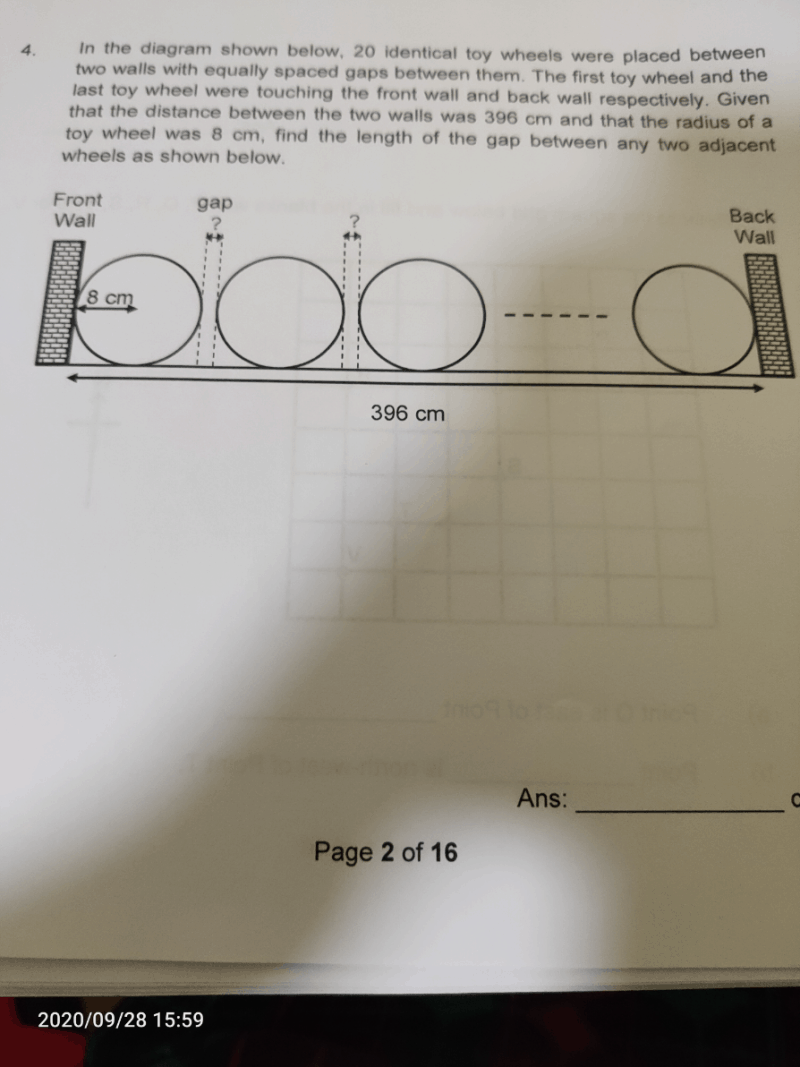# QuestionTotal no. of wheels = 20

Diameter of 1 wheel = 2 x 8cm = 16cm

Total length of space occupied all wheels = 20 x 16cm = 320cm

Total length of space occupied by gaps = 396cm – 320cm = 76cm

No. of gaps = 20 – 1 = 19

Length of 1 gap = 76cm ÷ 19 = 4cm

Hope you understand and all the best for your exams! :))

0 Replies 2 Likes